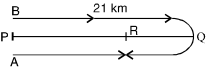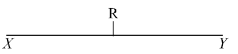## Speed, Time and Distance

#### Speed, Time and Distance

1. A man walks a distance of 35 kms. He walks for some time at 4 km per hour and for some time at 5 km per hr. If he walks at 5 km per hr. instead of 4 km per hr. and 4 km per hr. instead of 5 km per hr, he will walk 2 kms more in the same span of time. Find his total time of total journey.
1. 8.5 hours
2. 7.5 hours
3. 8 hours
4. 7 hours

1. Let the man walks for x hours at 4 km per hr. and y hours at 5 km per hr. and covers a distance of 35 kms.
∴  Distance = 4x + 5y = 35 ...(i)
Now, he walks at 5 km per hr.
for x hours and at 4 km per hr.
for y hours and covers a distance (35 + 2) = 37 kms
∴  Distance = 5x + 4y = 37...(ii)
By 5 × (i) – 4 × (ii) we have

 20x + 25y = 175 20x + 16y = 148 -       -       - ____________ 9y = 27

⇒  y = 3
Putting the value of (y) in equation (i), we have
4x + 5 × 3 = 35
⇒  4x = 35 – 15 = 20
⇒  x = 5
∴  Total time taken
= x + y = 5 + 3 = 8 hours.

##### Correct Option: C

Let the man walks for x hours at 4 km per hr. and y hours at 5 km per hr. and covers a distance of 35 kms.
∴  Distance = 4x + 5y = 35 ...(i)
Now, he walks at 5 km per hr.
for x hours and at 4 km per hr.
for y hours and covers a distance (35 + 2) = 37 kms
∴  Distance = 5x + 4y = 37...(ii)
By 5 × (i) – 4 × (ii) we have

 20x + 25y = 175 20x + 16y = 148 -       -       - ____________ 9y = 27

⇒  y = 3
Putting the value of (y) in equation (i), we have
4x + 5 × 3 = 35
⇒  4x = 35 – 15 = 20
⇒  x = 5
∴  Total time taken
= x + y = 5 + 3 = 8 hours.

1. Ram travelled one-third of a journey with a speed of 10 km per hr, the next one-third with a speed of 9 km per hr. and the rest at a speed of 8 km per hr. If he had travelled half the journey at speed of 10 km per hr. and the other half with a speed of 8 km per hr, he would have been 1 minute longer on the way. What distance did he travel?
1. 36 km
2. 32 km
3. 35 km
4. 40 km

1. Let the total distance travelled be x kms.
Case I :
Speed for the first one-third distance

 i.e. x kms =10 km per hr. 3

 ∴  Time taken = x hours 30

Similarly, time taken for the next one-third distance
 = x hours 27

and time taken for the last onethird distance
 = x hours. 24

∴  Total time taken to cover x kms.
 =x + x + xhours. 30 27 24

Case II :
Time taken for one-half distance at the speed of 10 km per hr.
 = x hrs. 20

and time taken for remaining 1/2 of distance
 = x hrs. at 8 km per hr. 16

 Total time taken =x + xhrs. 20 16

Time taken in (Case II – Case I)
 = 1 minute = 1 hr. 60

∴  According to the question
 x + x −x + x + x20 16 30 27 24

 = 1 60

 ⇒ 108x + 135x − 72x − 80x − 90x 2160

 = 1 60

 ⇒ 243x − 242x = 1 2160 60

 ⇒ x = 1 2160 60

 ⇒  x = 2160 = 36 km. 60

Hence the required distance
= 36 km.

##### Correct Option: A

Let the total distance travelled be x kms.
Case I :
Speed for the first one-third distance

 i.e. x kms =10 km per hr. 3

 ∴  Time taken = x hours 30

Similarly, time taken for the next one-third distance
 = x hours 27

and time taken for the last onethird distance
 = x hours. 24

∴  Total time taken to cover x kms.
 =x + x + xhours. 30 27 24

Case II :
Time taken for one-half distance at the speed of 10 km per hr.
 = x hrs. 20

and time taken for remaining 1/2 of distance
 = x hrs. at 8 km per hr. 16

 Total time taken =x + xhrs. 20 16

Time taken in (Case II – Case I)
 = 1 minute = 1 hr. 60

∴  According to the question
 x + x −x + x + x20 16 30 27 24

 = 1 60

 ⇒ 108x + 135x − 72x − 80x − 90x 2160

 = 1 60

 ⇒ 243x − 242x = 1 2160 60

 ⇒ x = 1 2160 60

 ⇒  x = 2160 = 36 km. 60

Hence the required distance
= 36 km.

1. Two men A and B start walking simultaneously from P to Q, a distance of 21 kms, at the speed of 3 km and 4 km an hour respectively. B reaches Q, returns immediately and meets A at R. Find the distance from P to R.
1. 22 km
2. 20 km
3. 16 km
4. 18 km

1. Let A and B meet after time t hours.Distance covered by A in t hours = 3 t km.
Distance covered by B in t hours = 4 t km.
Total distance covered by A and B = (3t + 4t) km = 7 t km.
From the diagram we can see that the total distance covered by A and B is equal to twice the distance between P and Q.
∴  7t = 2 × 21

 t = 2 × 21 = 6 hours 2

Distance PR = 6 × 3 = 18 km.

##### Correct Option: D

Let A and B meet after time t hours.Distance covered by A in t hours = 3 t km.
Distance covered by B in t hours = 4 t km.
Total distance covered by A and B = (3t + 4t) km = 7 t km.
From the diagram we can see that the total distance covered by A and B is equal to twice the distance between P and Q.
∴  7t = 2 × 21

 t = 2 × 21 = 6 hours 2

Distance PR = 6 × 3 = 18 km.

1. Two men A and B walk from X to Y a distance of 42 kms at 5 km and 7 km an hour respectively. B reaches Y and returns immediately and meets A at R. Find the distance from X to R.
1. 32 km
2. 30 km
3. 35 km
4. 40 km

1. When B meets A at R, by then B has walked a distance (XY + YR) and A, the distance XR.
That is both of them have together walked twice the distance from X to Y, i.e., 42 kms.Now, the ratio of speed of A and B is 5 : 7 and they walk 84 kms.
∴  Hence, the distance XR travelled by

 A = 5 × 84 = 35 kms. 5 + 7

##### Correct Option: C

When B meets A at R, by then B has walked a distance (XY + YR) and A, the distance XR.
That is both of them have together walked twice the distance from X to Y, i.e., 42 kms.Now, the ratio of speed of A and B is 5 : 7 and they walk 84 kms.
∴  Hence, the distance XR travelled by

 A = 5 × 84 = 35 kms. 5 + 7

1.  Walking at 3 of his usual speed a man is late by 4
 2 1 hours. The usual time would have been what? 2
1. 7 hours
2. 7.5 hours
3. 8 hours
4. 8.5 hours

1.  New speed is 3 of the usual speed 4

 ∴  New time taken = 4 of the usual time 3

 ∴ 4 of the usual time – Usual time = 5 3 2

 ⇒ 1 of the usual time = 5 3 2

 ∴ Usual time = 5 × 3 2

 = 15 hours or 7.5 hrs 2

##### Correct Option: B

 New speed is 3 of the usual speed 4

 ∴  New time taken = 4 of the usual time 3

 ∴ 4 of the usual time – Usual time = 5 3 2

 ⇒ 1 of the usual time = 5 3 2

 ∴ Usual time = 5 × 3 2

 = 15 hours or 7.5 hrs 2